# Texas Go Math Grade 2 Lesson 13.5 Answer Key Subtraction Situations

Refer to our Texas Go Math Grade 2 Answer Key Pdf to score good marks in the exams. Test yourself by practicing the problems from Texas Go Math Grade 2 Lesson 13.5 Answer Key Subtraction Situations.

## Texas Go Math Grade 2 Lesson 13.5 Answer Key Subtraction Situations

Unlock the Problem

Mrs. Baxter had 153 paintbrushes. She gave some of them to her students. Now she has 117 paintbrushes. How many did she give to her students?The number of paintbrushes did she give to her students is 36 paintbrushes.

Explanation:
Given that Mrs. Baxter had 153 paintbrushes and she gave some of them to her students. Now she has 117 paintbrushes, so the number of paintbrushes did she give to her students is 153 – 117 which is 36 paintbrushes.

What information am I given?
After giving some to students, she has _________ paintbrushes.

After giving some to students, she has 117 paintbrushes.

Plan

What is my plan or strategy?
I can _____________

Solve

Show how you solve the problem._____________ paintbrushes
The number of paintbrushes did she give to her students is 36 paintbrushes.

Explanation:
The number of paintbrushes did she give to her students is 36 paintbrushes.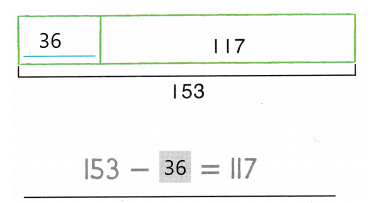HOME CONNECTION • Your child used o model and a number sentence to represent the problem. These help show what the missing amount is in order to solve the problem.

Try Another Problem

Label the model. Write a number sentence with afor the unknown number. Solve.

• What information am I given?
• What is my plan or strategy?

Texas Go Math Grade 2 Pdf Lesson 13.5 Answer Key Question 1.
Casey has a package of 250 stickers. He gives 107 stickers to his sister. How many stickers does Casey have now?___________ stickers
The number of stickers Casey has now is 143 stickers.

Explanation:
The given information is Casey has a package of 250 stickers and he gives 107 stickers to his sister, so the number of stickers Casey has now is 250 – 107 which is 143 stickers. Here, we have performed subtraction to know the number of stickers Casey has.Question 2.
Mr. Morton has a box of paper. After he takes 4-2 sheets of paper out of the box, there are 38 sheets of paper in the box. How many sheets of paper were in the box to start?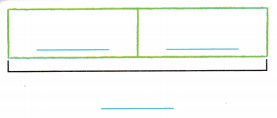___________ sheets of paper
The number of sheets of paper in the box to start is 42 sheets.

Explanation:
Given that Mr. Morton has a box of paper. After he takes 4-2 sheets of paper out of the box, there are 38 sheets of paper in the box. So the number of sheets of paper were in the box to start is 38 + 4 which is 42 sheets.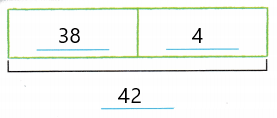Math Talk
Mathematical Processes

Explain how your number sentence shows the first problem.

Share and Show

Label the model. Write a number sentence with afor the unknown number. Solve.

There are 316 students at the school. If 120 students stay after school to play sports, how many students do not stay after school?__________ students
The number of students who do not stay after school is 196 students.

Explanation:
Given that there are 316 students at a school If 120 students stay after school to play sports. So the number of students who do not stay after school is 316 – 120 = 196 students.Problem Solving

Solve. Write or draw to show your work.

Question 4.
H.O.T. Rita has 250 pennies. Some pennies are in a box and some are in her bank. There are more than 100 pennies in each place. How many pennies could be in each place?___________ pennies in a box
___________ pennies in her bank
125 pennies in a box.
125 pennies in her bank.

Explanation:
Given that Rita has 250 pennies and some pennies are in a box and some are in her bank. There are more than 100 pennies in each place. So the number of pennies could be in each place is 250 ÷ 2 which is 125 pennies in a box and 125 pennies in her bank.

Question 5.
H.O.T. Multi-Step Mr. Taylor has 65 drawings in his classroom and 56 drawings ¡n the hail. 13 drawings are of pets. How many drawings are not of pets?
___________ drawings
The number of drawings are not of pets are 121.

Explanation:
Given that Mr. Taylor has 65 drawings in his classroom and 56 drawings ¡n the hail and 13 drawings are of pets. So the number of drawings are not of pets are 65+56 which is 121 are not pets.

Question 6.
Analyze There are 275 clocks on the shelves at The Clock Shop. Mr. Young puts 52 of those clocks on the walls. How many clocks are on the shelves now?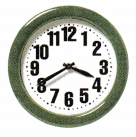(A) 223
(B) 327
(C) 213
B.

Explanation:
Given that there are 275 clocks on the shelves at the Clock Shop. Mr. Young puts 52 of those clocks on the walls, so the number of clocks are on the shelves now is 275+52 which is 327.

Joe’s first book has 84 pictures. It has 73 more pictures than his second book. How many pictures are in the second book?
Write a number sentence with afor the unknown number. Then solve.
____________ pictures
The number of pictures are in the second book is 157 pictures.

Explanation:
Given that Joe’s first book has 84 pictures and it has 73 more pictures than his second book. So the number of pictures are in the second book is 84 + 73 which is 157 pictures

Question 8.
Texas Test Prep There are 426 students at the school. Some students walk to school. 180 students take the bus to school. How many students walk to school?(A) 127
(B) 117
(C) 517
The number of students walk to school is 246 students.

Explanation:
Given that there are 426 students at the school and some students walk to school. 180 students take the bus to school, so the number of students walk to school is 426 – 180 which is 246 students.

TAKE HOME ACTIVITY • Have your child explain how he or she solved one of the problems in this lesson.

### Texas Go Math Grade 2 Lesson 13.5 Homework and Practice Answer Key

Label the model. Write a number sentence with afor the missing number. Solve.

Question 1.
There are 426 students at the school. Some students walk to school. 180 students take the bus to school. How many students walk to school?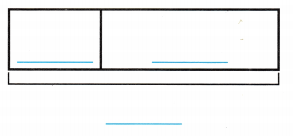_____________ students
The number of students walking to school is 246 students.

Explanation:
Given that there are 426 students at the school and some students walk to school. 180 students take the bus to school. So the number of students walking to school is 426 – 180 which is 246 students.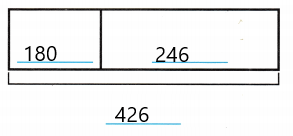Problem Solving

Solve. Write or draw to show your work.

Question 2.
Molly has 270 stickers. Some stickers are animal stickers and some are smiley stickers. There are more than 100 stickers of each kind. How many stickers of each kind could there be?
_____________ animal stickers
_____________ smiley stickers
135 animal stickers.
135 smiley stickers.

Explanation:
Given that Molly has 270 stickers and some stickers are animal stickers and some are smiley stickers. There are more than 100 stickers of each kind. So the number of stickers of each kind would be 270 ÷ 2 which is 135 stickers of animals and 135 stickers of smiley.

Question 3.
Multi-Step Mr Stevens has 48 books in one bookcase and 84 books in another. 15 books are mysteries. How many books are not mysteries?
_____________ books
The number of books that are not mysteries is 117 books.

Explanation:
Given that Mr. Stevens has 48 books in one bookcase and 84 books in another, so the number of books did Mr. Stevens had is 48 + 84 which is 132, and in that 15 books are mysteries. So the number of books that are not mysteries is 132 – 15 which is 117 books.

Lesson Check

Question 4.
There are a total of 446 sheep and goats at the Town Fair. There are 179 sheep. How many goats are there?
(A) 333
(B) 267
(C) 625
B.

Explanation:
Given that there are a total of 446 sheep and goats at the Town Fair and there are 179 sheep, so the number of goats are there is 446 – 179 which is 267 sheep.

Question 5.
There are 192 cameras at the store. 85 of the cameras are in boxes. How many cameras are not in boxes?
(A) 107
(B) 97
(C) 277
A.

Explanation:
Given that there are 192 cameras at a store and 85 of the cameras are in the boxes. So the number of cameras are not in the boxes is 192 – 85 which is 107 cameras.

There are 286 bikes in the warehouse. Then 124 bikes are taken to stores. How many bikes are still in the warehouse?
(A) 162
(B) 410
(C) 152
A.

Explanation:
Given that there are 286 bikes in a warehouse and then 124 bikes are taken to stores. So the number of bikes still in the warehouse is 286 – 124 which is 162 bikes.

Question 7.
There are 500 sheets of paper in a box. Teachers use some of the paper. Now there are 138 sheets of paper in the box. How many sheets of paper did the teachers use?
(A) 362
(B) 338
(C) 372